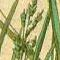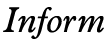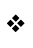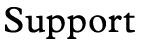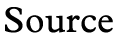Back to List

Plain
Coloured
Gaudy

This code
in plain text

### Browsing infix.h

InfixExamineP (lines 721-852)

```0721  [ InfixExamineP brief x a b w flag lines;
0722    switch(infix_term_type)
0723    {   INFIXTT_NUMBER:
0724            if (brief) "; == ", noun;
0725            print "; The number ", noun, " == \$", (InfixHex) noun;
0726            if (noun >= 32 && noun < 127) print " == '", (char) noun, "'";
0727            new_line;
0728        INFIXTT_NAMEDOBJECT:
0729            print "~", (name) noun, "~ (", noun, ")^"; if (brief) return;
0730            <<Showobj noun>>;
0731        INFIXTT_CONSTANT:
0732            if (brief) "; == ", noun;
0733            switch(infix_data1 & 15)
0734            {   nothing:
0735                    print "; Constant ", (InfixPrintConstant) infix_parsed_lvalue,
0736                    " == ", noun, "^";
0737                Object: <<Showobj noun>>;
0738                Class: print "Class ", (name) noun, "^";
0739                    objectloop (a ofclass noun)
0740                    {   if (flag) print ", "; else print "Contains: ";
0741                        print (name) a, " (", a, ")"; flag=true;
0742                    }
0743                    if (flag==false) "No object is of this class";
0744            }
0745            new_line;
0746        INFIXTT_ATTRIBUTE:
0747            if (brief) "; == ", noun;
0748            if (noun>=48 || noun<0) "; No such attribute";
0749            print "; Attribute ", (InfixPrintAttribute) noun,
0750                " (numbered ", noun, ")^";
0751            objectloop (x has noun)
0752            {   if (flag) print ", ";
0753                else print "Each of these ~has ", (InfixPrintAttribute) noun, "~: ";
0754                print (name) x, " (", x, ")"; flag = true;
0755            }
0756            if (flag == false) "No object ~has ", (InfixPrintAttribute) noun, "~";
0757            new_line;
0758        INFIXTT_PROPERTY:
0759            if (brief) "; == ", noun;
0760            print "; Property ", (property) noun, " (numbered ", noun, ")^";
0761            objectloop (x provides noun)
0762            {   if (flag) print ", "; else print "Provided by: ";
0763                print (name) x, " (", x, ")"; flag = true;
0764            }
0765            if (flag == false) "Which is not provided by any object";
0766            new_line;
0767        INFIXTT_DWORD:
0768            if (brief) "; == ", noun;
0769            if (noun == 0) "; This word is not in the dictionary";
0770            a = noun->#dict_par1;
0771            print "; Dictionary word '", (address) noun;
0772            if (a & 4) print "//p";
0773            print "' (address ", noun, ")";
0774            if (a)
0775            {   print ": ";
0776                if (a & 2) print "meta ";
0777                if (a & 1) print "verb   ";
0778                if (a & 8) print "preposition   ";
0779                if (a & 4) print "pluralising ";
0780                if (a & 128) print "noun ";
0781            }
0782            new_line;
0783            if (a & 1) <<Showverb noun>>;
0784        INFIXTT_ROUTINE:
0785            if (brief) "; == ", noun;
0786            print "; Routine ", (InfixPrintRoutine) infix_parsed_lvalue,
0787                " (number ", infix_parsed_lvalue, ",
0788                packed address ", noun, ")^";
0789        INFIXTT_GLOBAL:
0790            if (brief) "; == ", noun;
0791            print "; Global ", (InfixPrintGlobal) infix_parsed_lvalue,
0792                " == ", noun, "^";
0793        INFIXTT_ARRAY:
0794            if (brief) "; == ", noun;
0795            print "; Array ", (InfixPrintArray) infix_parsed_lvalue, " ";
0796            infix_data1 = infix_data1 % 16;
0797            switch(infix_data1)
0798            {   0: print "->"; a=0;
0799                1: print "-->"; a=0;
0800                2: print "string"; a=1;
0801                3: print "table"; a=1;
0802            }
0803            print " ", infix_data2, "^; == "; b=infix_data2; if (x) b++;
0804            for (w=b-1:w>=a:w--)
0805                if (infix_data1 == 0 or 2) { if (noun->w) break; }
0806                else { if (noun-->w) break; }
0807            if (b-1-w <= 5) w=b-1;
0808            for (:x<=w:x++)
0809                if (infix_data1 == 0 or 2) print noun->x, " ";
0810                else print noun-->x, " ";
0811            if (w<a) print "(", b-a, " zero entries)";
0812            else if (w<b-1) print "(then ", b-1-w, " zero entries)";
0813            new_line;
0814        INFIXTT_ACTION:
0815            if (brief) "; == ", noun;
0816            if (noun >= #lowest_fake_action_number
0817                && noun <= #highest_fake_action_number)
0818               "; Fake action ", (InfixPrintFakeAction) noun,
0819                " (numbered ", noun, ")^Is not generated by any grammar";
0820            print "; Action ", (InfixPrintAction) noun,
0821                " (numbered ", noun, ")^";
0822            w = 0-->4;
0823            for (b=0:b<(0-->4 + 5)-->0:b++)
0824            {   w = 0-->4 + 7 + b*9;
0825                if ((w->#dict_par1) & 1)
0826                {   a = (0-->7)-->(\$ff-(w->#dict_par2));
0827                    lines = a->0; a++;
0828                    for (:lines > 0:lines--)
0829                    {   a = UnpackGrammarLine(a);
0830                        if (action_to_be == noun)
0831                        {   print "'", (address) w, "' "; DebugGrammarLine();
0832                            new_line;
0833                            flag = true;
0834                        }
0835                    }
0836                }
0837            }
0838            if (flag == 0) "Is not generated by any grammar";
0839        INFIXTT_SYSFUN:
0840            if (brief) "; == ", noun;
0841           "; System function ~", (address) infix_parsed_lvalue, "~ has
0842            not been overridden by any routine and so has its standard
0843            definition.";
0844        INFIXTT_STATICSTRING:
0845            if (brief) "; == ", noun;
0846            if (metaclass(noun) ~= String) "; ", noun, " is not a string.";
0847            print "~", (string) noun, "~^";
0848        INFIXTT_LOGICAL:
0849            if (noun==true) "; true"; if (noun==false) "; false";
0850            "; ", noun;
0851    }
0852  ];
```

Last updated 27 February 2004. This site is no longer supported; information may be out of date.
Maintained as a historical archive by the Interactive Fiction Technology Foundation. Copyright 1993-2018 IFTF, CC-BY-SA unless otherwise noted.
This page was originally managed by Graham Nelson (graham@gnelson.demon.co.uk) assisted by C Knight.